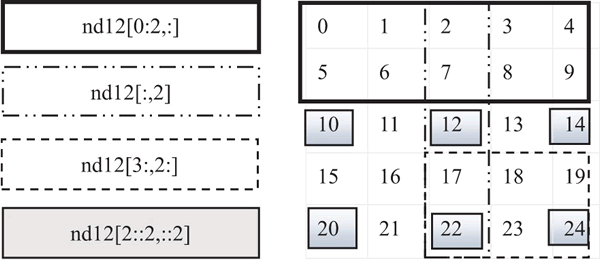# NumPy ndarray数组元素的获取

```import numpy as np

np.random.seed(2019)
nd1 = np.random.random()
#获取指定位置的数据, 获取第4个元素
nd1
#截取一段数据
nd1[3:6]
#截取固定间隔数据
nd1[1:6:2]
#倒序取数
nd1[::-2]
#截取一个多维数组的一个区域内数据
nd2=np.arange(25).reshape([5,5])
nd2[1:3,1:3]
#截取一个多维数组中, 数值在一个值域之内的数据
nd2[(nd2>3)&(nd2<10)]
#截取多维数组中, 指定的行,如读取第2,3行
nd2[[1,2]]  #或nd12[1:3,:]
##截取多维数组中, 指定的列,如读取第2,3列
nd2[:,1:3]``````import numpy as np
from numpy import random as nr

a=np.arange(1,25,dtype=float)
c1=nr.choice(a,size=(3,4))  #size指定输出数组形状
c2=nr.choice(a,size=(3,4),replace=False)  #replace缺省为True, 即可重复抽取。
#下式中参数p指定每个元素对应的抽取概率, 缺省为每个元素被抽取的概率相同。
c3=nr.choice(a,size=(3,4),p=a / np.sum(a))
print("随机可重复抽取")
print(c1)
print("随机但不重复抽取")
print(c2)
print("随机但按制度概率抽取")
print(c3)```

[[  7.  22.  19.  21.]
[  7.   5.   5.   5.]
[  7.   9.  22.  12.]]

[[ 21.   9.  15.   4.]
[ 23.   2.   3.   7.]
[ 13.   5.   6.   1.]]

[[ 15.  19.  24.   8.]
[  5.  22.   5.  14.]
[  3.  22.  13.  17.]]